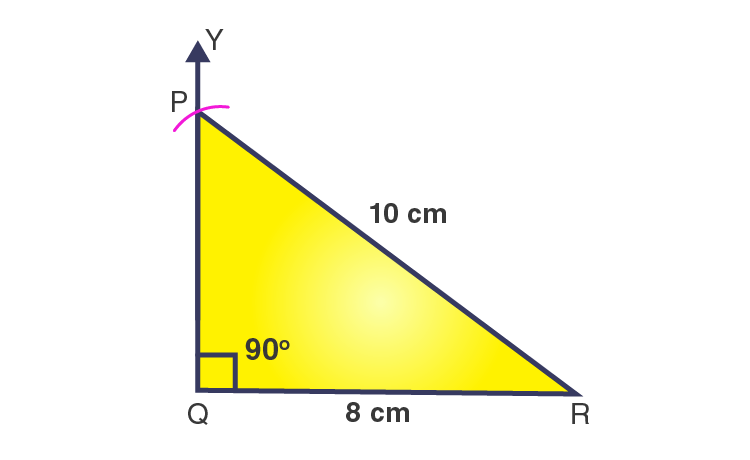# Construct the Construct the right angled ΔPQR, where m∠Q = 90°, QR = 8cm and PR = 10 cm.

SolutionThe steps for construction are given below:

1. Draw a line segment BC = 4 cm.

2. From point B, draw a ray BX to making an angle of 90o i.e. ∠XBC = 90o.

3. Considering C as a center and radius 6 cm, draw an arc that cuts the ray BX at A.

4. Join AC.

Then, ΔABC obtained is the required right angled triangle.(0)(0)